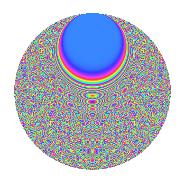# Properties

 Label 1575.2.crLevel 1575 Weight 2 Character orbit cr Rep. character $$\chi_{1575}(16,\cdot)$$ Character field $$\Q(\zeta_{15})$$ Dimension 1888 Sturm bound 480

# Learn more about

## Defining parameters

 Level: $$N$$ = $$1575 = 3^{2} \cdot 5^{2} \cdot 7$$ Weight: $$k$$ = $$2$$ Character orbit: $$[\chi]$$ = 1575.cr (of order $$15$$ and degree $$8$$) Character conductor: $$\operatorname{cond}(\chi)$$ = $$1575$$ Character field: $$\Q(\zeta_{15})$$ Sturm bound: $$480$$

## Dimensions

The following table gives the dimensions of various subspaces of $$M_{2}(1575, [\chi])$$.

Total New Old
Modular forms 1952 1952 0
Cusp forms 1888 1888 0
Eisenstein series 64 64 0

## Trace form

 $$1888q$$ $$\mathstrut +\mathstrut 3q^{2}$$ $$\mathstrut -\mathstrut 3q^{3}$$ $$\mathstrut +\mathstrut 235q^{4}$$ $$\mathstrut -\mathstrut 8q^{5}$$ $$\mathstrut -\mathstrut 8q^{6}$$ $$\mathstrut -\mathstrut 8q^{7}$$ $$\mathstrut -\mathstrut 16q^{8}$$ $$\mathstrut -\mathstrut 3q^{9}$$ $$\mathstrut +\mathstrut O(q^{10})$$ $$1888q$$ $$\mathstrut +\mathstrut 3q^{2}$$ $$\mathstrut -\mathstrut 3q^{3}$$ $$\mathstrut +\mathstrut 235q^{4}$$ $$\mathstrut -\mathstrut 8q^{5}$$ $$\mathstrut -\mathstrut 8q^{6}$$ $$\mathstrut -\mathstrut 8q^{7}$$ $$\mathstrut -\mathstrut 16q^{8}$$ $$\mathstrut -\mathstrut 3q^{9}$$ $$\mathstrut -\mathstrut 4q^{10}$$ $$\mathstrut -\mathstrut 6q^{11}$$ $$\mathstrut -\mathstrut 23q^{12}$$ $$\mathstrut -\mathstrut 6q^{13}$$ $$\mathstrut +\mathstrut 9q^{14}$$ $$\mathstrut -\mathstrut 42q^{15}$$ $$\mathstrut +\mathstrut 223q^{16}$$ $$\mathstrut -\mathstrut 12q^{17}$$ $$\mathstrut -\mathstrut 6q^{19}$$ $$\mathstrut -\mathstrut 2q^{20}$$ $$\mathstrut -\mathstrut 30q^{21}$$ $$\mathstrut +\mathstrut 2q^{22}$$ $$\mathstrut -\mathstrut 22q^{23}$$ $$\mathstrut -\mathstrut 26q^{24}$$ $$\mathstrut -\mathstrut 8q^{25}$$ $$\mathstrut +\mathstrut 76q^{26}$$ $$\mathstrut +\mathstrut 18q^{27}$$ $$\mathstrut -\mathstrut 20q^{28}$$ $$\mathstrut -\mathstrut 6q^{29}$$ $$\mathstrut +\mathstrut 25q^{30}$$ $$\mathstrut +\mathstrut 3q^{31}$$ $$\mathstrut +\mathstrut 4q^{32}$$ $$\mathstrut +\mathstrut 6q^{33}$$ $$\mathstrut -\mathstrut 10q^{34}$$ $$\mathstrut -\mathstrut 49q^{35}$$ $$\mathstrut +\mathstrut 20q^{36}$$ $$\mathstrut -\mathstrut 6q^{37}$$ $$\mathstrut -\mathstrut 22q^{38}$$ $$\mathstrut +\mathstrut 7q^{39}$$ $$\mathstrut -\mathstrut 22q^{40}$$ $$\mathstrut -\mathstrut 38q^{41}$$ $$\mathstrut -\mathstrut 26q^{42}$$ $$\mathstrut -\mathstrut 16q^{43}$$ $$\mathstrut +\mathstrut 6q^{44}$$ $$\mathstrut +\mathstrut 73q^{45}$$ $$\mathstrut +\mathstrut 2q^{46}$$ $$\mathstrut +\mathstrut 3q^{47}$$ $$\mathstrut +\mathstrut 44q^{48}$$ $$\mathstrut -\mathstrut 8q^{49}$$ $$\mathstrut -\mathstrut 28q^{50}$$ $$\mathstrut -\mathstrut 8q^{51}$$ $$\mathstrut -\mathstrut 14q^{52}$$ $$\mathstrut +\mathstrut 14q^{53}$$ $$\mathstrut -\mathstrut 11q^{54}$$ $$\mathstrut -\mathstrut 34q^{55}$$ $$\mathstrut -\mathstrut 48q^{56}$$ $$\mathstrut -\mathstrut 144q^{57}$$ $$\mathstrut -\mathstrut 46q^{58}$$ $$\mathstrut -\mathstrut 45q^{59}$$ $$\mathstrut +\mathstrut 53q^{60}$$ $$\mathstrut +\mathstrut 3q^{61}$$ $$\mathstrut -\mathstrut 52q^{62}$$ $$\mathstrut -\mathstrut 11q^{63}$$ $$\mathstrut -\mathstrut 436q^{64}$$ $$\mathstrut +\mathstrut 19q^{65}$$ $$\mathstrut +\mathstrut 5q^{66}$$ $$\mathstrut +\mathstrut 3q^{67}$$ $$\mathstrut -\mathstrut 256q^{68}$$ $$\mathstrut -\mathstrut 27q^{69}$$ $$\mathstrut +\mathstrut 8q^{70}$$ $$\mathstrut -\mathstrut 42q^{71}$$ $$\mathstrut -\mathstrut 15q^{72}$$ $$\mathstrut -\mathstrut 6q^{73}$$ $$\mathstrut -\mathstrut 32q^{74}$$ $$\mathstrut +\mathstrut 97q^{75}$$ $$\mathstrut -\mathstrut 16q^{76}$$ $$\mathstrut -\mathstrut 23q^{77}$$ $$\mathstrut +\mathstrut 40q^{78}$$ $$\mathstrut +\mathstrut 15q^{79}$$ $$\mathstrut +\mathstrut 94q^{80}$$ $$\mathstrut +\mathstrut q^{81}$$ $$\mathstrut -\mathstrut 20q^{82}$$ $$\mathstrut -\mathstrut 48q^{83}$$ $$\mathstrut -\mathstrut 125q^{84}$$ $$\mathstrut -\mathstrut 13q^{85}$$ $$\mathstrut -\mathstrut 22q^{86}$$ $$\mathstrut -\mathstrut 41q^{87}$$ $$\mathstrut -\mathstrut 22q^{88}$$ $$\mathstrut -\mathstrut 39q^{89}$$ $$\mathstrut -\mathstrut 178q^{90}$$ $$\mathstrut +\mathstrut 30q^{91}$$ $$\mathstrut -\mathstrut 30q^{92}$$ $$\mathstrut +\mathstrut 50q^{93}$$ $$\mathstrut -\mathstrut 13q^{94}$$ $$\mathstrut +\mathstrut 18q^{95}$$ $$\mathstrut +\mathstrut 23q^{96}$$ $$\mathstrut -\mathstrut 6q^{97}$$ $$\mathstrut -\mathstrut 142q^{98}$$ $$\mathstrut -\mathstrut 30q^{99}$$ $$\mathstrut +\mathstrut O(q^{100})$$

## Decomposition of $$S_{2}^{\mathrm{new}}(1575, [\chi])$$ into irreducible Hecke orbits

The newforms in this space have not yet been added to the LMFDB.